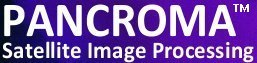See additional satellite image processing articles at www.PANCROMA.com menu selection 'White Papers'

# Satellite Image Deconvolution

Gaussian Low Pass Filter

(See the earlier article entitled FFT Image Analysis for background information on deconvolution).

We will start our discussion of satellite image deconvolution by talking about low pass filters. Low pass filters are essentially smoothing filters. Such a filter convolves a user defined Gaussian convolution kernel with the target image. The effect is to pass low frequencies and filter high frequencies from the image. This might be useful in improving a Landsat gap filled image for example if some residual image striping still exists.

In order to use the PANCROMA™ Gaussian smoothing utility, open a single band file by selecting 'File' | 'Open'. Then select 'FFT' | 'Image Fourier Analysis' | 'Gaussian Low Pass Filter'. A data entry box will become visible that allows you to input the Gaussian distribution parameters standard deviation and (if you wish) distribution amplitude. If the 'Un-Normalized Distribution' check box is not checked (default) then the Gaussian distribution will be normalized so that pixel brightnesses of the processed image will remain approximately the same as in the source image. If the box is checked, the convolution kernel will not be normalized.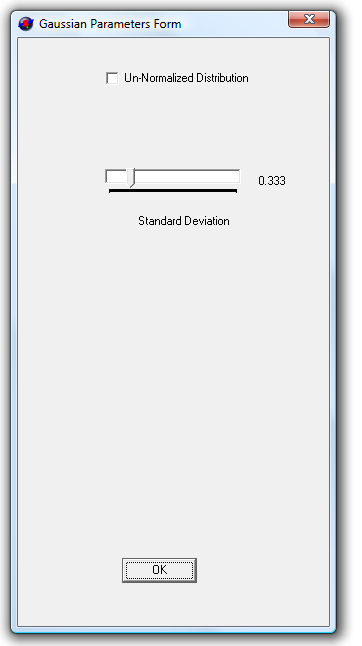Data Entry Box

Set the standard deviation and if desired the amplitude using the track bar sliders.

Inverse Gaussian Deconvolution

In general, low pass filters are not especially interesting for satellite image processing work except as an illustration of a more interesting topic: image deconvolution. The low pass filter will blur an image by convolving a Gaussian convolution kernel with the target image. It is generally possible to recover the image by deconvolving the blurred image with the same convolution kernel. In particular, the Fourier transformed band image is divided by the Fourier Transform of the convolution kernel instead of multiplying by the kernel as in convolution. The image is recovered by taking the inverse FFT of the product array. Theoretically, these are inverse operations and the original image can be completely recovered.

This can be seen in the following three images. The first one is the target image. The second image has been blurred using the low pass filter. The third image has been recovered by deconvolving with the same Gaussian convolution kernel that was used to create the blurred image, as described above.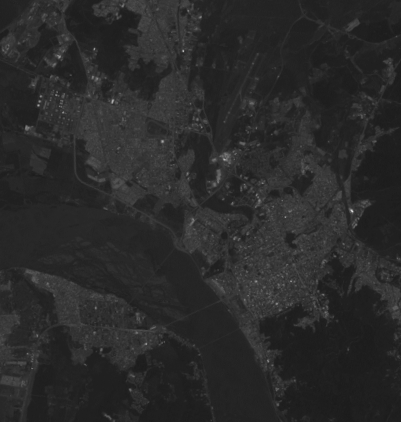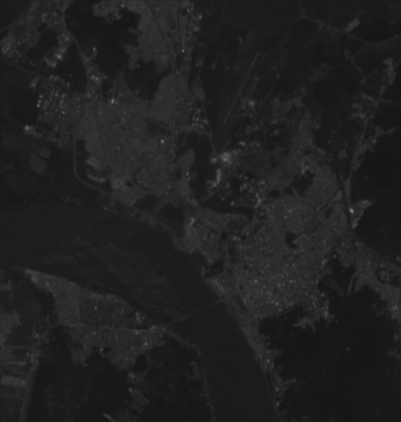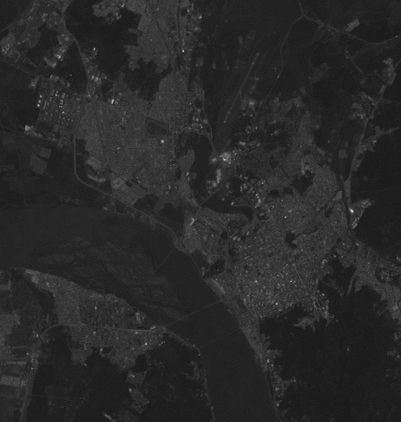Original band image, Gaussian blurred image, image recovered by deconvolution using the same Gaussian convolution kernel in an inverse operation.

IMPORTANT NOTE: In reality, the convolution process may not be easily reversible. Inverse convolution operations have many potential issues

• The inversion process is usually very sensitive to small perturbations.

• The inversion process is always corrupted by rounding errors (noise).

• Instead of the desired solution we often see the amplified noise only.

• If the transformed convolution matrix is close to singular, we can easily lose important information.

In addition, the FFT does not necessarily transform an image from the spatial domain to the frequency domain with complete fidelity. In particular, certain image features, combined with certain convolution kernel characteristics can cause the reverse FFT of the product array to "ring". If one attempts to reverse the convolution process (deconvolution), the convoluted array does not correspond to the input array, and the deconvoluted array will be corrupted. If sigma for example is too large, the deconvolution may "blow up" into an incomprehensible noisy mess as shown in the image below. However, if sigma is not too large, the inversion generally works for most areas of the image. Image discontinuities like shorelines for example may still exhibit ringing effects.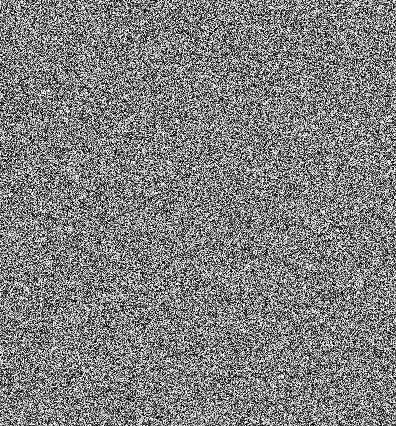Noise amplification resulting from overdriving a deconvolution.

An over-driven deconvolution can be remedied by using a noise filter as explained below.

Image Deconvolution

Taking our convolution/deconvolution concept a step further, we might ask "What happens if we attempt to deconvolve an image that has not been deliberately blurred" (by us) but which has become blurred by other means? Satellite images can be blurry for a variety of reasons, including lens distortion, CCD sensor distortion, atmospheric turbulence, and many other reasons. If we knew the nature of the distortion we might recover the image using the same technique described above, using the appropriate convolution kernel to deconvolve the image. The distortion is typically described by something called the Point Spread Function, which as its name suggests describes how a point source of light actually ends up getting displayed in our image. Rather than a point mapping to a single pixel, it is actually spread among many pixels. Deconvolving with the Point Spread Function can recover the correct value for each pixel by recovering the portions that have been spread among all the other pixels in the image and inserting them back into the target pixel.

Unfortunately, the Point Spread Function is often unknown. However the Gaussian, Moffat and Exponential distributions offered by PANCROMA™ can approximate it in many cases. Deconvolving with a reasonable PSF distribution with the right parameters can often improve an image. The "right" parameters being selected by trial and error.

The following two images illustrate the technique. The first image is uncorrected. The second image has been deconvolved with a normalized Gaussian distribution with sigma=0.5. The sigma value was determined by trying different values until the focus of the image seemed optimal by visual inspection. Generally, larger values for sigma result in a more pronounced sharpening effect, until the image is over-sharpened resulting in first in excessive image noise, followed by the image "blowing up" into an unrecognizably noisy mess. In fact the onset of image noise is apparent in the sharpened image at the bottom of the next page.

PANCROMA™ offers two deconvolution utilities: Fixed Kernel Size and Variable Kernel Size, and three PSF distributions, Gaussian, Moffat and Exponential. The deconvolution kernel defines the extents of the selected distribution used to deconvolve the image. The fixed kernel size is five, meaning that the distribution is truncated at +/- 2 pixels from the pixel being operated on. Such a kernel for a normalized Gaussian distribution with sigma=1 is shown in the table below. (The kernel is centered on the (2, 2) position with the partitioning shown.)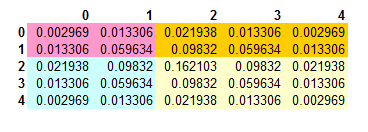Fixed Gaussian kernal of size = 5.

In order to deconvolve using fixed kernel size open a single grayscale band file and select: "FFT" | "Image Fourier Analysis" | "Gaussian Deconvolution" | "Fixed Kernel Size = 5". You can also increase the kernel size in odd increments up to 29. In order to do so, select "FFT" | "Image Fourier Analysis" | "Gaussian Deconvolution" | "Variable Kernel Size". The fixed kernel size runs faster than the variable size and takes less RAM. It generally works well for low values of sigma (<1).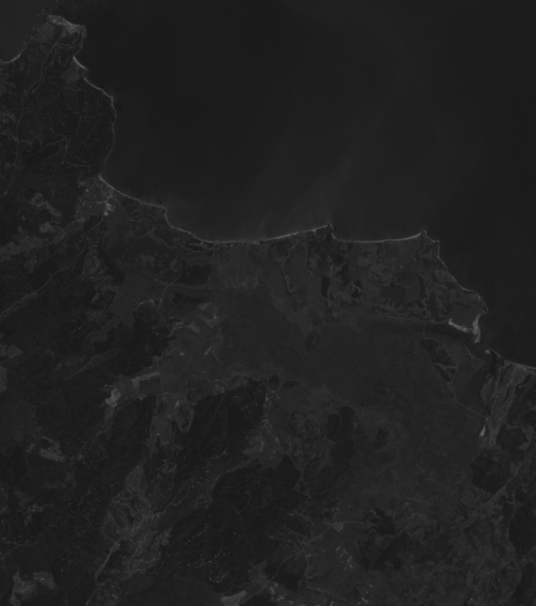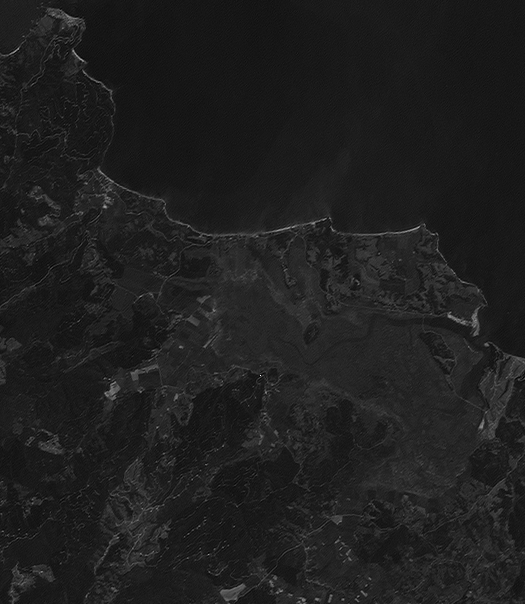Original band image on the left, deconvoluted using the PANCROMA™ Gaussian Point Spread Function on the right. The effect is dramatic but typical, especially for the shorter wavelength bands.

If the deconvolution causes the image to "blow up" as described above, it is usually beneficial to use a noise filter. The over driven image problem lies in the division by the FFT of the deconvolution kernel. The kernel can have a lot of small values as a result of transformed noise. When a pixel in the transformed image is divided by one of these small values, an extremely large value results, resulting in an over-driven image. This can be remedied by adjusting the thresholding value. The default value is 0.01. If you have a noisy image you can increase this value, increasing the effect of the noise filter.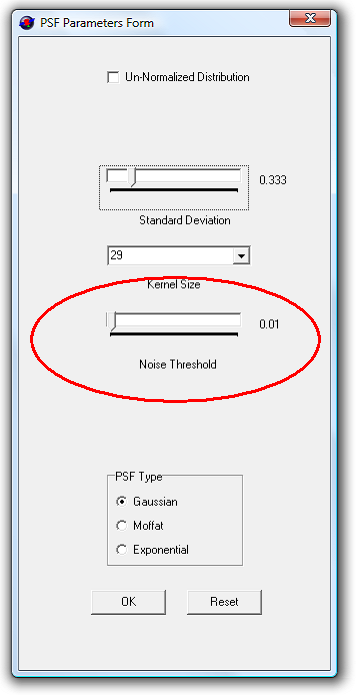Filter threshold setting.

Increasing the filter setting filters out more noise. However the valid data is also affected to some degree so keep the filter as low as possible.

In order to use the Moffat distribution, use the same procedure as outlined above except selecting the 'Moffat' PSF type on the Gaussian Parameters Form. When you do, the 'Beta' track bar will become visible.

The Moffat distribution has two use-adjustable parameters: the standard deviation (sigma) and the beta coefficient. Increasing sigma and decreasing beta will increase the deblurring effect, while possibly causing increasing noise. The thresholding track bar works as described above to filter noisy images.

In general the Moffat PSF seems to work better for many images as it allows more control oft the shape of the PSF, allowing a closer match with the actual PSF and in turn better results.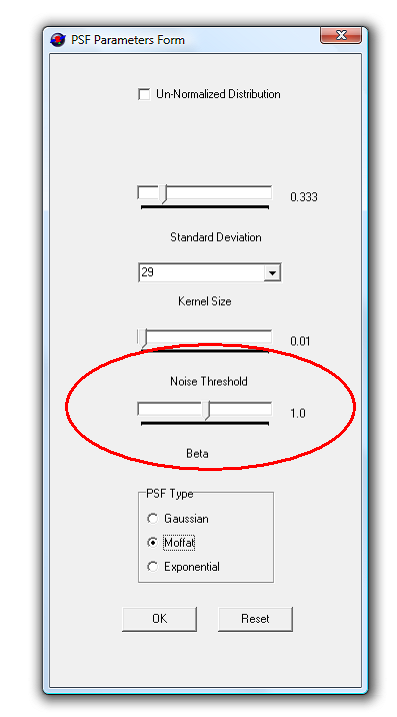Beta parameter (wing) setting.

IMPORTANT NOTE: The maximum size of input files for PANCROMA™ Fourier operations is 100MB. This means that files the size of the Landsat panchromatic band are too big. The reason is that Fourier operations require large floating point data structures that use a lot of memory. If you want to deconvolve large images like Landsat panchromatic bands, you will have to subset your image. However PANCROMA™ should easily handle Landsat multispectral bands.

Why Deconvolution?

Deconvolution is somewhat related to image sharpening techniques such as unsharp mask. The advantage of deconvolution is that, unlike unsharp mask filters the deconvolution kernel can be adjusted by the user to closely match the Point Spread Function. This should result in a higher quality image sharpening process.

The Huang paper entitled Impact of sensor’s point spread function on land cover characterization: assessment and deconvolution describes the MODIS Point Spread Function. The MODIS PSF is essentially a Gaussian distribution with sigma=123.5m or approximatly half the MODIS pixel resolution of 250m. The USGS paper entitled Landsat 7 on-orbit modulation transfer function estimation has some information regarding the Landsat PSF. Although it is mainly aimed at measuring the Modulation Transfer Function (which is an alternative method for measuring optical fidelity), some of the information regarding the System Transfer Function and the Point Spread Function may be useful in estimating Gaussian approximation parameters for the Landsat PSF.